## 1-D Multisignal Analysis

This section takes you through the features of 1-D multisignal wavelet analysis, compression and denoising using the Wavelet Toolbox™ software. The rationale for each topic is the same as in the 1-D single signal case.

The toolbox provides the following functions for multisignal analysis.

Analysis-Decomposition and Synthesis-Reconstruction Functions

Function Name

Purpose

`mdwtdec`

Multisignal wavelet decomposition

`mdwtrec`

Multisignal wavelet reconstruction and extraction of approximation and detail coefficients

Decomposition Structure Utilities

Function Name

Purpose

`chgwdeccfs`

Change multisignal 1-D decomposition coefficients

`wdecenergy`

Multisignal 1-D decomposition energy repartition

Compression and Denoising Functions

Function Name

Purpose

`mswcmp`

Multisignal 1-D compression using wavelets

`mswcmpscr`

Multisignal 1-D wavelet compression scores

`mswcmptp`

Multisignal 1-D compression thresholds and performance

`wdenoise`

Wavelet signal denoising

`mswthresh`

Perform multisignal 1-D thresholding

### 1-D Multisignal Analysis

Load an image file, and display its dimensions. For purposes of this example, unless stated otherwise, you will treat the data as a multisignal. Each row of the image is one signal.

```load thinker size(X)```
```ans = 1×2 192 96 ```

Plot some signals from the data.

```plot(X(1:5,:)',"r") hold on plot(X(21:25,:)',"b") plot(X(31:35,:)',"g") hold off xlim([1 96]) grid on```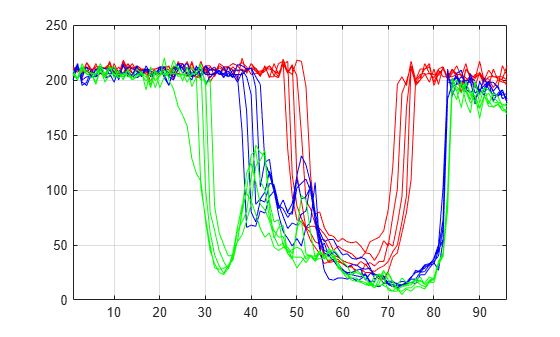Decomposition and Reconstruction

Use `mdwtdec` to perform a wavelet decomposition of the multisignal at level 2 using the `db2` wavelet.

`dec = mdwtdec("r",X,2,"db2")`
```dec = struct with fields: dirDec: 'r' level: 2 wname: 'db2' dwtFilters: [1x1 struct] dwtEXTM: 'sym' dwtShift: 0 dataSize: [192 96] ca: [192x26 double] cd: {[192x49 double] [192x26 double]} ```

Use `chgwdeccfs` to generate a new wavelet decomposition structure from `dec`. For each signal, change the wavelet coefficients by setting all the coefficients of the detail of level 1 to zero.

`decBIS = chgwdeccfs(dec,"cd",0,1);`

Use `mdwtrec` to perform a wavelet reconstruction of the multisignal. Plot some of the new signals.

```Xbis = mdwtrec(decBIS); plot(Xbis(1:5,:)',"r") hold on plot(Xbis(21:25,:)',"b") plot(Xbis(31:35,:)',"g") hold off grid on xlim([1 96])```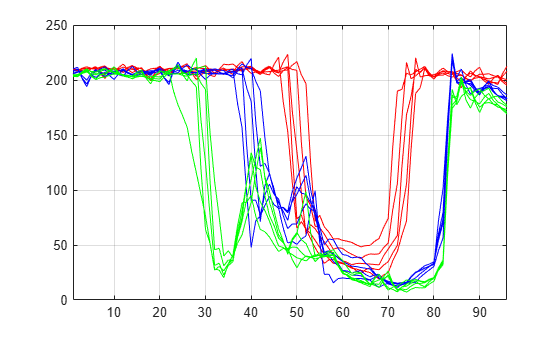Compare old and new signals by plotting them together.

```idxSIG = [1 31]; plot(X(idxSIG,:)',"r",LineWidth=2) hold on plot(Xbis(idxSIG,:)',"b",Linewidth=2) hold off grid on xlim([1 96])```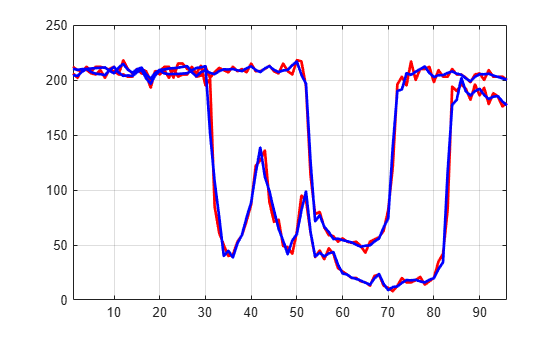Set the wavelet coefficients at level 1 and 2 for signals 31 to 35 to the value zero, perform a wavelet reconstruction of signal 31, and compare some of the old and new signals.

```decTER = chgwdeccfs(dec,'cd',0,1:2,31:35); Y = mdwtrec(decTER,'a',0,31); figure plot(X([1 31],:)',"r",LineWidth=2) hold on plot([Xbis(1,:); Y]',"b",LineWidth=2) hold off grid on xlim([1 96])```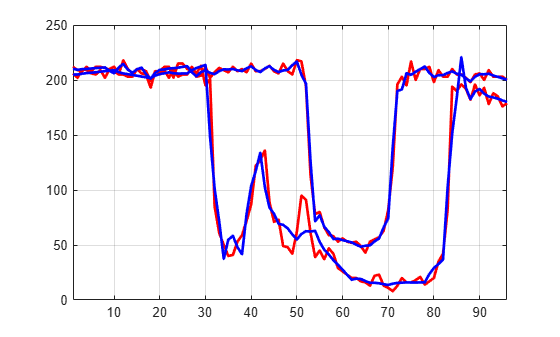Use `wdecenergy` to compute the energy of signals and the percentage of energy for wavelet components. Display the energy of two of the signals.

```[E,PEC,PECFS] = wdecenergy(dec); Ener_1_31 = E([1 31])```
```Ener_1_31 = 2×1 106 × 3.7534 2.2411 ```

Compute the percentage of energy for wavelet components of signals 1 and 31. The first column shows the percentage of energy for approximations at level 2. Columns 2 and 3 show the percentage of energy for details at level 2 and 1, respectively.

`PEC_1_31 = PEC([1 31],:) `
```PEC_1_31 = 2×3 99.7760 0.1718 0.0522 99.3850 0.2926 0.3225 ```

Display the percentage of energy for wavelet coefficients of signals 1 and 31. As we can see in the `dec` structure, there are 26 coefficients for the approximation and the detail at level 2, and 49 coefficients for the detail at level 1.

```PECFS_1 = PECFS(1,:); PECFS_31 = PECFS(31,:); plot(PECFS_1,"r",LineWidth=2) hold on plot(PECFS_31,"b",LineWidth=2) hold off grid on xlim([1 size(PECFS,2)])```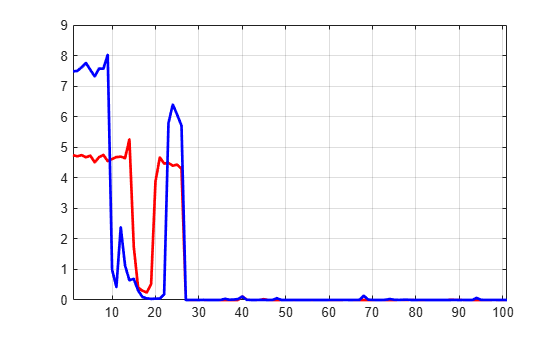Compression

Use `mswcmp` to compress the signals to obtain a percentage of zeros near 95% for the wavelet coefficients.

```[XC,decCMP,~] = mswcmp("cmp",dec,"N0_perf",95); [Ecmp,PECcmp,PECFScmp] = wdecenergy(decCMP);```

Plot the original signals 1 and 31, and the corresponding compressed signals.

```plot(X([1 31],:)',"r",LineWidth=2) hold on plot(XC([1 31],:)',"b",LineWidth=2) hold off grid on xlim([1 96])```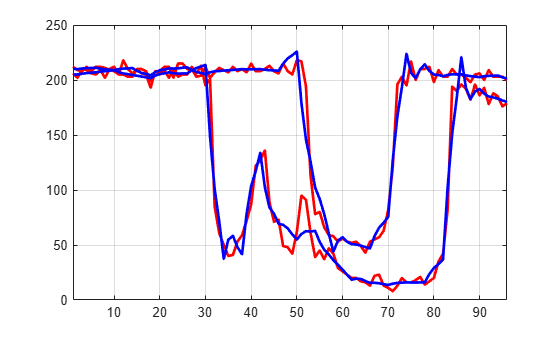Use `mswcmptp` to compute thresholds, percentage of energy preserved and percentage of zeros associated with the `L2_perf` method preserving at least 95% of energy.

```[THR_VAL,L2_Perf,N0_Perf] = mswcmptp(dec,"L2_perf",95); idxSIG = [1,31]; Thr = THR_VAL(idxSIG)```
```Thr = 2×1 256.1914 158.6085 ```
`L2per = L2_Perf(idxSIG) `
```L2per = 2×1 96.5488 94.7197 ```
`N0per = N0_Perf(idxSIG) `
```N0per = 2×1 79.2079 86.1386 ```

Compress the signals to obtain a percentage of zeros near 60% for the wavelet coefficients.

`[XC,decCMP,~] = mswcmp("cmp",dec,"N0_perf",60);`

`XC` signals are the compressed versions of the original signals in the row direction. Compress the `XC` signals in the column direction

`XX = mswcmp("cmpsig","c",XC,"db2",2,"N0_perf",60);`

Plot original signals `X` and the compressed signals `XX` as images.

```tiledlayout(1,2) nexttile image(X) nexttile image(XX) colormap(pink(222))```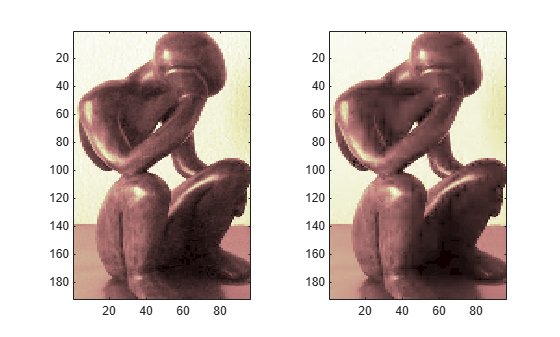Denoising

You can use the `wdenoise` function to denoise a multisignal. By default, `wdenoise` denoises the columns of matrix. Denoise the multisignal using `wdenoise` with default settings. Compare with the original signals.

```XD = wdenoise(X'); XD = XD'; figure plot(X([1 31],:)',"r",LineWidth=2) hold on plot(XD([1 31],:)',"b",Linewidth=2) hold off grid on xlim([1 96])```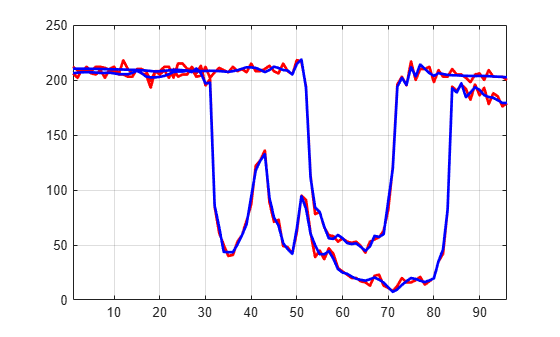Denoeud, L., Garreta, H., and A. Guénoche. "Comparison of Distance Indices Between Partitions." In International Symposium on Applied Stochastic Models and Data Analysis, 432–440. Brest, France: École Nationale des Télécommunications de Bretagne, 2005.